# engNRICH

##### Age 16 to 18 Challenge Level:

Welcome to engNRICH: the engineering section of stemNRICH. This contains mathematical activities for students aged 16 - 19 designed to complement and enhance the study of engineering.

Area of maths Style Question Description
Dynamics See also the dynamics problems on the physNRICH pages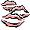Model Solutions How do these modelling assumptions affect the solutions?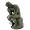Dam Busters 1 At what positions and speeds can the bomb be dropped to destroy the dam?Ramping it Up Look at the calculus behind the simple act of a car going over a step.Dam Busters 2 Can you work out which of the equations models a bouncing bomb? Will you be able to hit the target?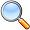Making More Tracks Given the equation for the path followed by the back wheel of a bike, can you solve to find the equation followed by the front wheel?
Structural engineering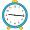Overarch 1 This short question asks if you can work out the most precarious way to balance four tiles.Bridge Builder In this short problem we investigate the tensions and compressions in a framework made from springs and ropes.More Bridge Building Which parts of these framework bridges are in tension and which parts are in compression?Overarch 2 What is the furthest a tower can theoretically arch over?Beam Me Up Look at the mathematics of the bending of beams.Euler's Buckling Formula Find the critial load at which a beam will buckle.
Digital circuits and logicProcedure Solver Can you think like a computer and work out what this flow diagram does?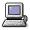Adding Machine Can you set the logic gates so that this machine can decide how many bulbs have been switched on?Not Another NAND! Prove that you can make any type of logic gate using just NAND gates.Circular Circuitry What will happen when you switch on these circular circuits?
Decisions, logistics and controlMaximum Flow Given the graph of a supply network and the maximum capacity for flow in each section find the maximum flow across the network.Pattern Recognition What is a random pattern?
Mechanical engineeringBuilding up Friction A series of activities to build up intuition on the mathematics of frictionStonehenge Explain why, when moving heavy objects on rollers, the object moves twice as fast as the rollers. Try a similar experiment yourself.Stonehenge Is Going Nowhere See why extensions of the ideas of the log rolling in Stonehenge would lead to a lack of progress in activity!
Power, work and energy in engineering See also the Power, Work and Energy problems on the physNRICH pagesExplore the power of aeroplanes, spaceships and horses.Go Spaceship Go Show that even this powerful spaceship will eventually run out of overtaking power.Turbo Turbines
A look at power generation using wind turbines.
Fluid mechanics See the Fluids Problems on the physNRICH pages
Electrical EngineeringThe Wheatstone Bridge Explore the mathematics behind the famous Wheatstone Bridge circuit.A Circuit Problem Find the voltages and currents in this interesting circuit configuration.Battery Modelling Find out how to model a battery mathematically.Differential Electricity As a capacitor discharges, its charge changes continuously. Find the differential equation governing this variation.Impedance Can Be Complex! Put your complex numbers and calculus to the test in this impedance calculation.
ArticlesAC/DC Circuits Find out how Ohm's law develops and find a fundamental link to complex numbers along the way.Who Is an Engineer? This short article gives a quick perspective of engineering after one year of a university engineering course and will be useful to help to understand what exactly goes on during an engineering degree course.